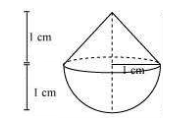# A solid is in the shape of a cone standing on a hemisphere with both their radii being equal to 1 cm and the height of the cone is equal to its radius. Find the volume of the solid in terms of π.Given

r = 1 cm

h = 1 cm.

Find out

We have to find out the volume of the solid in terms of π

Solution

Total Volume = Volume of cone + Volume of Hemisphere

We know that

Volume of cone = 1/3 πr2h

Volume of hemisphere = 2/3πr3

= 1/3πr2h + 2/3πr3

= 1/3 πr2(h+2r)

= 1/3 × π × 1 × 1 × (1+2)

= π cm3

The volume of solid in terms of π is π cm3